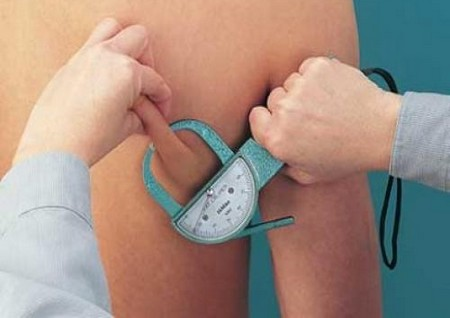# How To Calculate Body Fat Percentage

Calculating body fat percentage is essential to managing weight loss. It can be a bit of a challenge. However, a correct body fat percentage allows you to track you progress when you are actively seeking to lose weight. The way to calculate body fat percentage is different according to sex. Men biologically have less body fat than women. There calculations are different and we will review them both. Here are some quick and easy steps to help you calculate your personal body fat percentage.

Things you’ll need:

• Fabric tape measure
• Calculator

Men’s Calculations

Step 1

Weigh yourself. Try to weigh yourself early in the day. As the day goes on we tend to weigh a little more due to the effects of gravity. Take your weight and multiply it by 1.082. Add 94.420 and this should give you your first number. Label that number ‘factor 1’.Step 2

Take the fabric tape measure and measure your waist line. Multiply this number by 4.150. This is ‘factor 2’.

Step 3

Subtract factor 1 and factor 2. This is your lean body weight.

Step 4

Subtract you total body weight and lean body weight. This number is your body fat weight.

Step 5

Multiply your body fat weight by 100 and divide that by your total body weight. This is your body fat percentage.

Women’s Calculations

Step 1

Weight yourself. Take your total body weight and multiply it by .732. Add 8.987. Label this number factor 1.

Step 2

Measure your wrist with your fabric tape measure. Divide this number by 3.140. Label this number factor 2.

Step 3

Measure your abdomen. Multiply this number by 0.157. Label this number factor 3.

Step 4

Measure your hips. Multiply this number by .249. Label this number factor 4.

Step 5

Measure your forearm. Multiply this number by .434. Label this number factor 5.

Step 6

Follow this formula. Factor 1 + Factor 2- Factor 3- Factor 4+ Factor 5. This is a woman’s lean body mass calculation.

Step 7

Subtract total body weight and lean body mass. This is your body fat weight.

Step 8

Take your body fat weight and multiply it by 100. Then divide by the total body weight. This number is the body fat percentage for a female.

Step 9

Keep track of your weight decreases and increases. Write them down in a notebook so that you can monitor your own progress. Make a habit of checking your body fat percentage every two weeks. Try to measure and weight yourself at the same time each day with the same tools. This method should be used in full every time you decide to calculate your body fat percentage.

Filed Under: Health & Personal CareAbout the Author: Andrew Reinert is a health care professional who loves to share different tips on health and personal care. He is a regular contributor to MegaHowTo and lives in Canada.